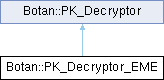Botan 3.0.0-alpha0 Crypto and TLS for C&
Botan::PK_Decryptor_EME Class Referencefinal

`#include <pubkey.h>`

Inheritance diagram for Botan::PK_Decryptor_EME:## Public Member Functions

template<typename Alloc >
secure_vector< uint8_t > decrypt (const std::vector< uint8_t, Alloc > &in) const

secure_vector< uint8_t > decrypt (const uint8_t in[], size_t length) const

secure_vector< uint8_t > decrypt_or_random (const uint8_t in[], size_t length, size_t expected_pt_len, RandomNumberGenerator &rng) const

secure_vector< uint8_t > decrypt_or_random (const uint8_t in[], size_t length, size_t expected_pt_len, RandomNumberGenerator &rng, const uint8_t required_content_bytes[], const uint8_t required_content_offsets[], size_t required_contents) const

PK_Decryptor_EMEoperator= (const PK_Decryptor_EME &)=delete

PK_Decryptor_EMEoperator= (PK_Decryptor_EME &&)=delete

PK_Decryptor_EME (const PK_Decryptor_EME &)=delete

PK_Decryptor_EME (const Private_Key &key, RandomNumberGenerator &rng, const std::string &eme, const std::string &provider="")

PK_Decryptor_EME (PK_Decryptor_EME &&)=delete

size_t plaintext_length (size_t ptext_len) const override

~PK_Decryptor_EME ()

## Detailed Description

Decryption with an MR algorithm and an EME.

Definition at line 539 of file pubkey.h.

## ◆ PK_Decryptor_EME() [1/3]

 Botan::PK_Decryptor_EME::PK_Decryptor_EME ( const Private_Key & key, RandomNumberGenerator & rng, const std::string & eme, const std::string & provider = `""` )

Construct an instance.

Parameters
 key the key to use inside the decryptor rng the random generator to use eme the EME to use provider the provider to use

Definition at line 115 of file pubkey.cpp.

119 {
120 m_op = key.create_decryption_op(rng, padding, provider);
121 if(!m_op)
122 throw Invalid_Argument("Key type " + key.algo_name() + " does not support decryption");
123 }

## ◆ ~PK_Decryptor_EME()

 Botan::PK_Decryptor_EME::~PK_Decryptor_EME ( )
default

## ◆ PK_Decryptor_EME() [2/3]

 Botan::PK_Decryptor_EME::PK_Decryptor_EME ( const PK_Decryptor_EME & )
delete

## ◆ PK_Decryptor_EME() [3/3]

 Botan::PK_Decryptor_EME::PK_Decryptor_EME ( PK_Decryptor_EME && )
delete

## ◆ decrypt() [1/2]

template<typename Alloc >
 secure_vector< uint8_t > Botan::PK_Decryptor::decrypt ( const std::vector< uint8_t, Alloc > & in ) const
inlineinherited

Same as above, but taking a vector

Parameters
 in the ciphertext
Returns
decrypted message

Definition at line 101 of file pubkey.h.

102 {
103 return decrypt(in.data(), in.size());
104 }
secure_vector< uint8_t > decrypt(const uint8_t in[], size_t length) const
Definition: pubkey.cpp:17

References Botan::CryptoBox::decrypt().

## ◆ decrypt() [2/2]

 secure_vector< uint8_t > Botan::PK_Decryptor::decrypt ( const uint8_t in[], size_t length ) const
inherited

Decrypt a ciphertext, throwing an exception if the input seems to be invalid (eg due to an accidental or malicious error in the ciphertext).

Parameters
 in the ciphertext as a byte array length the length of the above byte array
Returns
decrypted message

Definition at line 17 of file pubkey.cpp.

18 {
20
21 secure_vector<uint8_t> decoded = do_decrypt(valid_mask, in, length);
22
24 throw Decoding_Error("Invalid public key ciphertext, cannot decrypt");
25
26 return decoded;
27 }

Referenced by Botan::KeyPair::encryption_consistency_check().

## ◆ decrypt_or_random() [1/2]

 secure_vector< uint8_t > Botan::PK_Decryptor::decrypt_or_random ( const uint8_t in[], size_t length, size_t expected_pt_len, RandomNumberGenerator & rng ) const
inherited

Decrypt a ciphertext. If the ciphertext is invalid (eg due to invalid padding) or is not the expected length, instead returns a random string of the expected length. Use to avoid oracle attacks, especially against PKCS #1 v1.5 decryption.

Definition at line 78 of file pubkey.cpp.

82 {
83 return decrypt_or_random(in, length, expected_pt_len, rng,
84 nullptr, nullptr, 0);
85 }
secure_vector< uint8_t > decrypt_or_random(const uint8_t in[], size_t length, size_t expected_pt_len, RandomNumberGenerator &rng) const
Definition: pubkey.cpp:78

References Botan::PK_Decryptor::decrypt_or_random().

## ◆ decrypt_or_random() [2/2]

 secure_vector< uint8_t > Botan::PK_Decryptor::decrypt_or_random ( const uint8_t in[], size_t length, size_t expected_pt_len, RandomNumberGenerator & rng, const uint8_t required_content_bytes[], const uint8_t required_content_offsets[], size_t required_contents ) const
inherited

Decrypt a ciphertext. If the ciphertext is invalid (eg due to invalid padding) or is not the expected length, instead returns a random string of the expected length. Use to avoid oracle attacks, especially against PKCS #1 v1.5 decryption.

Additionally checks (also in const time) that: contents[required_content_offsets[i]] == required_content_bytes[i] for 0 <= i < required_contents

Used for example in TLS, which encodes the client version in the content bytes: if there is any timing variation the version check can be used as an oracle to recover the key.

Definition at line 30 of file pubkey.cpp.

37 {
38 const secure_vector<uint8_t> fake_pms = rng.random_vec(expected_pt_len);
39
40 uint8_t decrypt_valid = 0;
41 secure_vector<uint8_t> decoded = do_decrypt(decrypt_valid, in, length);
42
45
46 decoded.resize(expected_pt_len);
47
48 for(size_t i = 0; i != required_contents_length; ++i)
49 {
50 /*
51 These values are chosen by the application and for TLS are constants,
52 so this early failure via assert is fine since we know 0,1 < 48
53
54 If there is a protocol that has content checks on the key where
55 the expected offsets are controllable by the attacker this could
56 still leak.
57
58 Alternately could always reduce the offset modulo the length?
59 */
60
61 const uint8_t exp = required_content_bytes[i];
62 const uint8_t off = required_content_offsets[i];
63
64 BOTAN_ASSERT(off < expected_pt_len, "Offset in range of plaintext");
65
66 auto eq = CT::Mask<uint8_t>::is_equal(decoded[off], exp);
67
69 }
70
73
74 return decoded;
75 }
Definition: assert.h:54
static Mask< T > is_equal(T x, T y)
Definition: ct_utils.h:147
static Mask< T > is_zero(T x)
Definition: ct_utils.h:139

## ◆ operator=() [1/2]

 PK_Decryptor_EME & Botan::PK_Decryptor_EME::operator= ( const PK_Decryptor_EME & )
delete

## ◆ operator=() [2/2]

 PK_Decryptor_EME & Botan::PK_Decryptor_EME::operator= ( PK_Decryptor_EME && )
delete

## ◆ plaintext_length()

 size_t Botan::PK_Decryptor_EME::plaintext_length ( size_t ctext_len ) const
overridevirtual

Return an upper bound on the plaintext length for a particular ciphertext input length

Implements Botan::PK_Decryptor.

Definition at line 127 of file pubkey.cpp.

128 {
129 return m_op->plaintext_length(ctext_len);
130 }

The documentation for this class was generated from the following files: